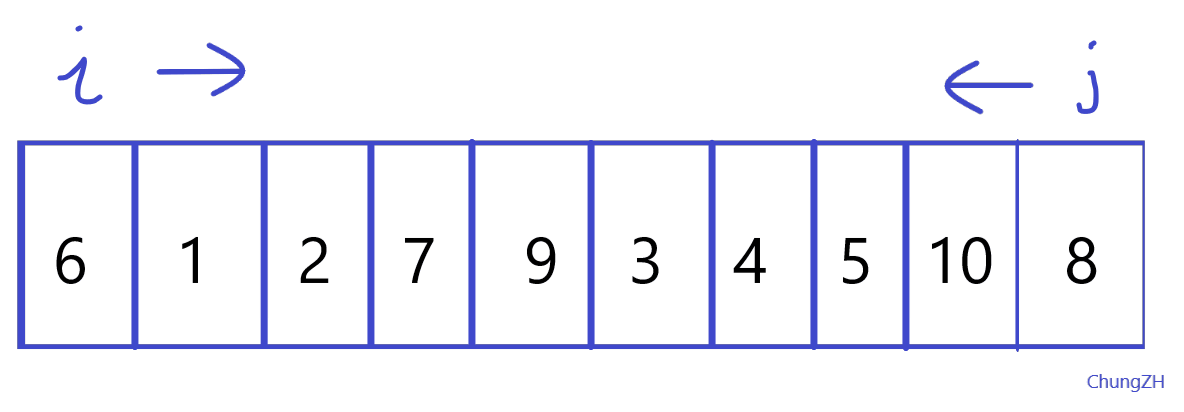``````#include <iostream>
#include <algorithm>
using namespace std;
int a;                             // 由于这个数组需要在函数中使用，所以定义为全局变量
void quicksort(int left, int right);    // 定义快速排序函数
int main()
{
int n;
cin >> n;
for (int i = 1; i <= n; i++)
{
cin >> a[i];
}

quicksort(1, n);

for (int i = 1; i <= n; i++)
{
cout << a[i] << " ";
}
return 0;
}

void quicksort(int left, int right)
{
int i, j;
int temp;
if (left > right)
return;

temp = a[left];                     // 基准数
i = left; j = right;                // 两个哨兵

while (i != j)                      // 两个哨兵一碰面就退出循环
{
while (a[j] >= temp && i < j)   // 哨兵j 向左寻找比基准数小的数
{
j--;
}
while (a[i] <= temp && i < j)   // 哨兵i 向右寻找比基准数大的数
{
i++;
}

// 如果哨兵i和哨兵j还没碰面，就把两个哨兵指向的元素交换。
if (i < j)
{
swap(a[i], a[j]);
}
}

// 最后将哨兵i和基准数交换
a[left]=a[i];
a[i] = temp;

quicksort(left, i-1);    // 继续处理左边的（递归）
quicksort(i+1, right);   // 继续处理右边的（递归）
return;

}
``````

（代码比桶排序的长多了。。。）

``````10
6 1 2 7 9 3 4 5 10 8
``````

``````1 2 3 4 5 6 7 8 9 10
``````

``````6 1 2 7 9 3 4 5 10 8
3 1 2 5 4 6 9 7 10 8
2 1 3 5 4 6 9 7 10 8
1 2 3 5 4 6 9 7 10 8
1 2 3 5 4 6 9 7 10 8
1 2 3 4 5 6 9 7 10 8
1 2 3 4 5 6 9 7 10 8
1 2 3 4 5 6 8 7 9 10
1 2 3 4 5 6 7 8 9 10
1 2 3 4 5 6 7 8 9 10
1 2 3 4 5 6 7 8 9 10
``````